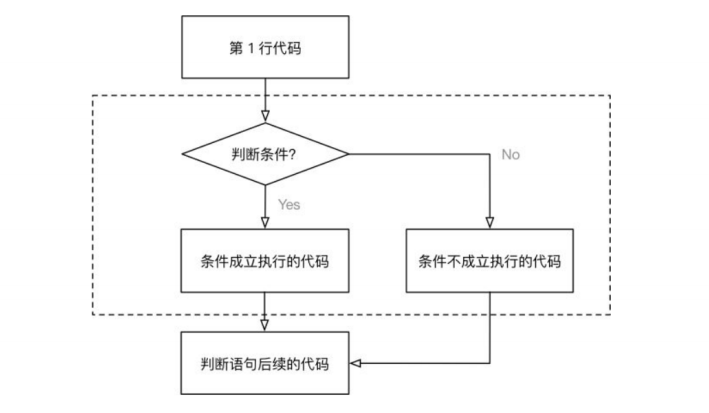# Python 条件语句怎么用

• 2022 年 8 月 29 日
湖南
• 本文字数：1596 字

阅读完需：约 5 分钟

## if 条件判断语句

python 语句是按固定顺序执行的，先执行前面的语句，再执行后面的语句。如果你像要程序按照你自己定制的流程执行，就需要用到流程控制的语句，最主要用到的是条件语句和循环语句。null

image-20210907141048465

if 用户名和密码正确:      登录成功else:      重新输入用户名和密码

if 语句的语法可以表示为：

if expression:      statement1else:      statement2

if expression:      statement1

if expression: statement1

score = 88if score > 80:      print("良好")else:      print("一般")

score = 70if score > 80:      print("良好")elif 60 < score <= 80:      print("还行")else:      print("不及格")

score = 70if score > 60:      print("不错哦")elif 60 < score <= 80:      print("还好")else:      print("不及格")

score = 70if score > 60:      print("不错哦")if 60 < score <= 80:      print("还好")else:      print("不及格")

## 条件语句嵌套

score = 88age = 1if score > 80:    print("良好")if age < 6:    print("神童")else:    print("一般")

## 你可能不知道的条件操作

a = ''if not a:      print("字符串为空")  if a:      print("字符串不为空")

if a == '':      print("字符串为空")if len(a) == 0:      print("字符串为空")

if a is None:      print("a 为 None")

if a == 0:      print("a 为 0")

## python 条件判断语句案例

name = ''password = ''if name == 'admin' and password == '123456':      print("登录成功")else:      print("登录失败")### 和牛

python程序员，wx号：shoubian01

## 评论